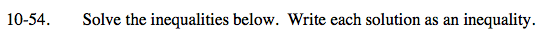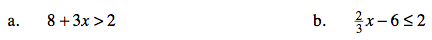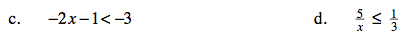### Home > CAAC > Chapter 10 > Lesson 10.2.1 > Problem10-54

10-54.Change the inequality to an equation and solve for x.
8 + 3x = 2
3x = −6
x = −2

Test both sides of the boundary point.
8 + 3(−3) > 2 8 + 3(−1) > 2
8 − 9 > 2 8 − 3 > 2
−1 > 2 5 > 2
False True

x > −2

Follow the steps in part (a).

x ≤ 12Follow the steps in part (a).

Follow the steps in part (a).

x ≥ 15